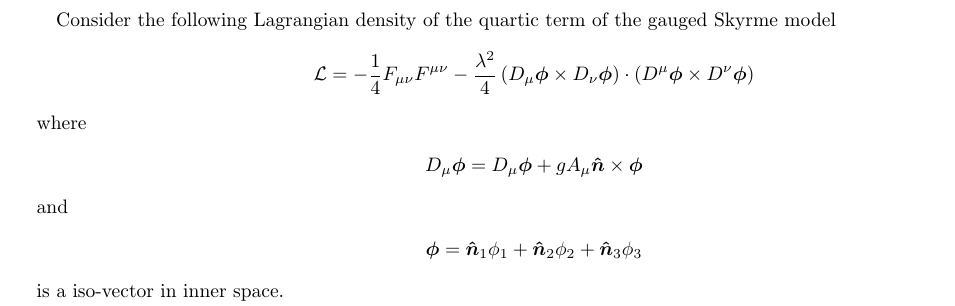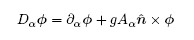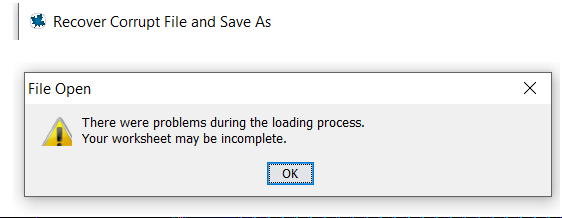## Simple non-commutative Differential Operator...

I'm using the differential operator D to differentiate variables and look at the structure of the equations, i.e. turn things like

Du-vt+v^2=0 into

D[1,2](u)-D(v)*t+v*D(t)+2*v*D(v)=0

However, it is assumed that this is a commutative operator, i.e. D[1,2]=D[2,1]. Can I use an option to have it not commute, or should I look into using the DETools package?

Thanks,

Mike

## How calculate the Skyrme of motion from the Lagran...

How can I calculate the Euler-Lagrange equation from the Lagrangian density from Gauged Baby Skyrme model in maple using the physics package? Here, the rules of the operation in the inner space are the same that of the vectors conventional: dot and cross products, etc...
Following below the Lagrangian density.Errata:## Error, (in simplify/power) unable to evaluate sign...

If I used factor, it didn't simplify to 0. As you may notice that this value should be 0.Hello,

every time I input a formula I get:

Typesetting:-mparsed(x^2 +5 -2,x^2+3; "_noterminate")

I can't get rid of this error: this is very basic, what happened?

TIA, Roberto

## Sockets package issues - Serial interfacing ...

I get the following errors when attempting to use the Sockets package to interface with the serial input and output for a USB device connected and reported to have no known problems by Windows 10:

with(Sockets):
LookupService("busboy");
Error, could not determine determine port number for service "busboy"

LookupService(998);

Errror, cannot  determine "tcp"  service on port 998

server :=
proc (sid)
Sockets:-Write(sid, sprintf("Hello %s on port %d, from %s\r\n", Sockets:-GetPeerHost(sid), Sockets:-GetPeerPort(sid), Sockets:-GetHostName()))
end proc;

Sockets:-Serve(GetPeerPort(sid), server);

Error, (in Sockets:-GetPeerHost) Unknown error

sid := Open("localhost", "echo");

Sockets:-Serve(GetPeerPort(sid), server);

Error, (in Sockets:-Serve) cannot bind address: Unknown error

## Solving the following PDE...

How can we solve the following pde by Maple?where v is velocity, v with dot is acceleration. (So, I think we will assume that acceleration is fixed.) And \delta is Dirac distribution.  E,I,m, M , g are fixed numbers.

Boundary conditions are:Initial conditions are:You can find the equation in the code: question.mw

## Corrupted file ...

Hi

I have a problem with my maple.corrupt file

Is there anybody who can help me solve this problem.

Casper

## how i can Substituting......

i want to gain diff(p(t), t) and diff(q(t), t) and Jacobian matrix
according to the attached pdf file.

thanks

 >(1)
 >(2)
 >(3)
 >(4)
 >(5)
 >(6)
 >(7)
 >## How do I run coding with goto on Maple? ...

Hi, I'm using Maple 2018 and I tried to run coding from https://www.maplesoft.com/applications/view.aspx?sid=4194&view=html

however, it said : unable to parse. I figured out that the problem maybe is in the if loop. though it seems perfectly fine, but it has some goto commands that i cannot search on maple website. does this mean that the goto cannot be used here and should be replaced? if yes, then how?

i am still learning on how to use maple. any help would be much appreciated. thank you!

this is the coding for if loop:

label_7;

rv:=vector([p1(x1pt,x2pt),p2(x1pt,x2pt)]):

numgeval:=numgeval+1;

printf("%5d (%8.4f,%8.4f)",numIter,rv,rv);

max:=n;

mg:=convert(sqrt(dotprod(rv,rv)),float);

printf("%12.4f",mg);

if(mg<tol or numIter>=max) then

goto(label_6);

else

numIter:=numIter+1;

fi;

v1:=x1pt+t*rv;

v2:=x2pt+t*rv;

newt:=evalf(subs({x1=v1,x2=v2},f1));

numfeval:=numfeval+1;

lam:=fsolve(diff(newt,t)=0,t,maxsols=1);

nv1:=evalf(subs({t=lam},v1));

nv2:=evalf(subs({t=lam},v2));

printf(" (%8.4f,%8.4f)%13.4f\n",x1pt,x2pt,lam);

x1pt:=nv1;

x2pt:=nv2;

goto(label_7);

label_6;

printf("\n\n-----------------------------------------");

printf("---------------------------------------------");

printf("\n\n Approximate Solution: ");

printf(" (%8.4f,%8.4f)\n",x1pt,x2pt);

Fvalue:=evalf(subs(x1=x1pt,x2=x2pt,f));

printf(" Maximum Functional Value: ");

printf("%21.4f",Fvalue);

printf("%22d",numgeval);

printf("\n Number function evaluations:");

printf("%22d",numfeval);

printf("\n\n-----------------------------------------");

printf("---------------------------------------------");

end:

## Can someone explain what DESol mean as a solution ...

Hello,

I am fairly new to using the Maple software, so I apologize if my question is completely idiotic. Apologies, also, because I could not manage to enter my code as code. When I pressed the button it made the whole text as a code.

I run the following code to seek -if there are any- analytic solutions for the following differential equation.

odeplus := (r^2+L^2)^(5/2)*(diff(f(r), \$(r, 2)))+((15/4)*r*(r^2+L^2)^(1/2)+3*(r^2+L^2)^(5/2)/r)*(diff(f(r), r))+M^2*f(r)/(r^2+L^2)^(5/2)-((5/2)*((r^2+L^2)^(1/2))(l-1)+(55/64)*r^2/(r^2+L^2)^(3/2)+(r^2+L^2)^(5/2)*(l^2+3*l+3/2)/r^2)*f(r)+(((r^2+L^2)^(1/2))(5+(5/2)*l)+(5/8)*r^2/(r^2+L^2)^(3/2)-(r^2+L^2)^(5/2)*(3/2+l)/r^2)*f(r) = 0

and then I do

dsolve(odeplus, f(r))

The solutions that Maple returns is given in terms of DESol. Could anyone try and break it down for me? What is this telling me and if I can indeed from the output obtain analytic solutions? Is this some sort of operator acting on something?

## simplify an equation ...

hi.

According to the fhgure attaceh how i can gain the equation (2-27) . I write the equation (2-26) in maple but I couldnot to gain that result.

If possible to reach equation via maple?

Thanks(1)(2)## Strange Behavior of DynamicSystems[BodePlot]...

I tried the example in BodePlot help.

restart;
with(DynamicSystems):
sys := TransferFunction( 1/(s-10) ):
BodePlot(sys);

That works OK. But, if I invoke Syrup, the example no longer works.

restart;
with(Syrup);
with(DynamicSystems):
ckt := [V, Rsrc(50), C1(15e-9), L1(15e-6), C2(22e-9), L2(15e-6), C3(22e-9), L3(15e-6), C4(15e-9), 1, Rload(50)];

sys := TransferFunction(TF);

BodePlot(sys);
I get a message "not a valid plot structure".  OK, try the example, again.

sys := TransferFunction( 1/(s-10) ):
BodePlot(sys);
I also get the "not a valid plot structure" message.

What am I doing wrong?

## How to i solve this complex integration in Maple?...

Respected sir,

I have attached a file that contain integration . Sir I am unable to solve please give me some hint.

1. I want to get final expression , want to get the value of constant from boundary condition.

2.I want to get the final expression (.......+c1)

3.I will put boundary condition to determine the value of c1

## Euler-method of forces on an airplane...

Struggling a bit with the Euler-method from task d) and further.## Fracsym package...

When i am running a code in maple worksheet , one error is shown by maple. My code and error (in bold) is below

Instructional workheet for the FracSym package
G. F. Jefferson and J. Carminati

Read in accompanying packages: ASP, DESOLVII and initialise using the with command:

read ASP v4.6.3.txt:

DESOLVII_V5R5 (March 2011)(c), by Dr. K. T. Vu, Dr. J. Carminati and Miss G.

Jefferson

The authors kindly request that this software be referenced, if it is used

in work eventuating in a publication, by citing the article:
K.T. Vu, G.F. Jefferson, J. Carminati, Finding generalised symmetries of

differential equations
using the MAPLE package DESOLVII,Comput. Phys. Commun. 183 (2012) 1044-1054.

-------------
ASP (November 2011), by Miss G. Jefferson and Dr. J. Carminati

The authors kindly request that this software be referenced, if it is used

in work eventuating in a publication, by citing the article:
G.F. Jefferson, J. Carminati, ASP: Automated Symbolic Computation of

Approximate Symmetries
of Differential Equations, Comput. Phys. Comm. 184 (2013) 1045-1063.

with(ASP);
[ApproximateSymmetry, applygenerator, commutator]
with(desolv);
[classify, comtab, defeqn, deteq_split, extgenerator, gendef, genvec,

icde_cons, liesolve, mod_eq, originalVar, pdesolv, reduceVar, reduceVargen,

symmetry, varchange]

Read in FracSym and initialise using the with command:
read FracSym.v1.16.txt;
FracSym (April 2013), by Miss G. Jefferson and Dr. J. Carminati

The authors kindly request that this software be referenced, if it is used

in work eventuating in a publication, by citing:
G.F. Jefferson, J. Carminati, FracSym: Automated symbolic computation of Lie

symmetries
of fractional differential equations, Comput. Phys. Comm. Submitted May 2013.

with(FracSym);
[Rfracdiff, TotalD, applyFracgen, evalTotalD, expandsum, fracDet, fracGen,

split]

BASIC OPERATORS

The Riemann-Liouville fractional derivatives is expressed in "inert" form using the FracSym routine Rfracdiff.
The explicit formula for the form of these fractional derivatives may be found in I. Podlubny, Fractional differential equations: An introduction to fractional derivatives, fractional differential equations, some methods of their solution and some of their applications, San Diego, 1999.)

Rfracdiff(u(x, t),t,alpha);
alpha
D[t     ](u(x, t))

If the fractional derivative is taken for a product, the generalised Leibnitz rule is used to express the result (the product operator used is &* and is non-commutative).
Rfracdiff(u(x, t)&*v(x,t),t,alpha);
infinity
-----
\
)                          (alpha - n)              n
/     binomial(alpha, n) D[t           ](u(x, t)) D[t ](v(x, t))
-----
n = 0
Rfracdiff(v(x, t)&*u(x,t),t,alpha);
infinity
-----
\
)                          (alpha - n)              n
/     binomial(alpha, n) D[t           ](v(x, t)) D[t ](u(x, t))
-----
n = 0

Fractional derivatives of integer order revert to the MAPLE diff routine.

Rfracdiff(u(x, t)&*v(x,t),t,2);
/ d  / d         \\             / d         \ / d         \
|--- |--- u(x, t)|| v(x, t) + 2 |--- u(x, t)| |--- v(x, t)|
\ dt \ dt        //             \ dt        / \ dt        /

/ d  / d         \\
+ u(x, t) |--- |--- v(x, t)||
\ dt \ dt        //

The FracSym rouine TotalD may also be used to find total derivatives. evalTotalD is then used to evaluate the result (in jet notation). For example,

TotalD(xi[x](x, y),x,2);
2
D[x ](xi[x](x, y))
evalTotalD([%],[y],[x]);
[     / d             \      2 / d  / d             \\
[y_xx |--- xi[x](x, y)| + y_x  |--- |--- xi[x](x, y)||
[     \ dy            /        \ dy \ dy            //

/ d  / d             \\       / d  / d             \\]
+ 2 |--- |--- xi[x](x, y)|| y_x + |--- |--- xi[x](x, y)||]
\ dy \ dx            //       \ dx \ dx            //]

EXAMPLE -  FINDING SYMMETRIES FOR A FRACTIONAL DE

Consider the fractional PDE from: R. Sahadevan, T. Bakkyaraj, Invariant analysis of time fractional generalized Burgers and Korteweg-de Vries equations, J. Math. Anal. Appl. 393 (2012) 341-347.

We use the Rfracdiff routine to express the
alpha
fractional derivative with respect to t:
fde1:=Rfracdiff(u(x, t),t,alpha) = (diff(u(x, t), x,x))+n*(u(x, t))^p*(diff(u(x, t),  x));
alpha             / d  / d         \\            p / d         \
D[t     ](u(x, t)) = |--- |--- u(x, t)|| + n u(x, t)  |--- u(x, t)|
\ dx \ dx        //              \ dx        /

sys1:=[Rfracdiff(u(x, t),t,alpha) = (diff(v(x, t), x)), Rfracdiff(v(x, t),t,alpha) = -u(x, t)*diff(u(x, t),x)];
[   alpha              d              alpha                      / d         \]
[D[t     ](u(x, t)) = --- v(x, t), D[t     ](v(x, t)) = -u(x, t) |--- u(x, t)|]
[                      dx                                        \ dx        /]

We use the the FracSym routine fracDet to find the determining equations for the symmetry for fde1.
NOTE: The fourth argument (some integer at least 1) corresponds to the number of terms to be "peeled off" from the sums which occur in the extended infintesimal function for the fractional derivative. A value of 2 provides a good balance between information for solution of determining equations and speed.

deteqs:=fracDet([sys1], [u, v],[x, t], 2, alpha=(0.1)..1);
Error, (in desolv/PickLHSDerivative) Cannot pick out the left hand side derivatives

Please suggest what problem it may be?

﻿